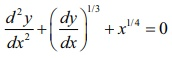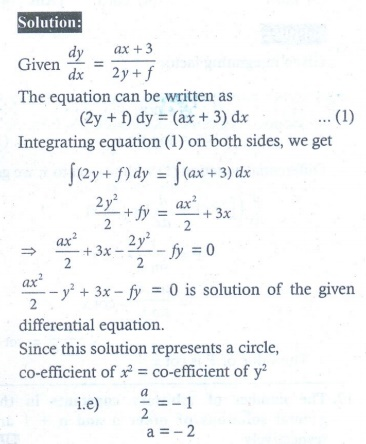Home | | Maths 12th Std | Exercise 10.9: Choose the correct answer

# Exercise 10.9: Choose the correct answer

Choose the correct or the most suitable answer from the given four alternatives - Maths Book back answers and solution for Exercise questions - Mathematics : Ordinary Differential Equations

EXERCISE 10.9

Choose the correct or the most suitable answer from the given four alternatives :

1. The order and degree of the differential equationare respectively

(1) 2, 3

(2) 3, 3

(3) 2, 6

(4) 2, 42. The differential equation representing the family of curves y = A cos(x + B), where A and B are parameters, is3. The order and degree of the differential equation √sin x dx + dy ) = √cos x dx − dy) is

(1) 1, 2

(2) 2, 2

(3) 1, 1

(4) 2, 14. The order of the differential equation of all circles with centre at ( h, k ) and radius ‘a’ is

(1) 2

(2) 3

(3) 4

(4) 15. The differential equation of the family of curves y = Aex + Bex , where A and B are arbitrary constants is6. The general solution of the differential equationis

(1) xy = k

(2) y = k log x

(3) y = kx

(4) log y kx7. The solution of the differential equation 2x dy/dx − y = 3 represents

(1) straight lines

(2) circles

(3) parabola

(4) ellipse8. The solution of dy/dx + p ( x) y = 0 is9. The integrating factor of the differential equationis

(1) x/eλ

(2) eλ/x

(3) λex

(4) ex10. The integrating factor of the differential equation dy/ dx + P ( x) y = Q(x) is x, then P(x)

(1) x

(2) x2/2

(3) 1/x

(4) 1/x211. The degree of the differential equationis

(1) 2

(2) 3

(3) 1

(3) 412. If p and q are the order and degree of the differential equationwhen

(1) p < q

(2) p = q

(3) p > q

(4) p exists and q does not exist13. The solution of the differential equationis

(1) y + sin−1 x = c

(2) x + sin−1 y = 0

(3) y2 + 2 sin−1 x = C

(4) x2 + 2 sin−1 y = 014. The solution of the differential equation dy/dx = 2xy is

(1) y = Cex2

(2) y = 2x2 + C

(3) y = Ce −x2 + C

(4) y = x2 + C15. The general solution of the differential equation= x + y is

(1) ex + ey = C

(2) ex + ey = C

(3) ex + ey = C

(4) ex + ey = C16. The solution ofis

(1) 2x + 2y = C

(2) 2x − 2y = C −

(3) 1/2x – 1/2y = C

(4) x + y = C17. The solution of the differential equationis18. If sin x is the integrating factor of the linear differential equation dy/dx+ Py = Q , then P is

(1) log sin x

(2) cos x

(3) tan x

(4) cot x19. The number of arbitrary constants in the general solutions of order n and n +1are respectively

(1) n −1, n

(2) n, n +1

(3) + 1, n + 2

(4) n +1, n20. The number of arbitrary constants in the particular solution of a differential equation of third order is

(1) 3

(2) 2

(3) 1

(4) 0

21. Integrating factor of the differential equationis

(1) 1 / x +1

(2) x +1

(3) 1 /  √(+1)

(4) √(+1)22.The population P in any year t is such that the rate of increase in the population is proportional to the population. Then

(1) P = Cekt

(2) P = Ce−kt

(3) P = Ckt

(4) P = C23. P is the amount of certain substance left in after time t. If the rate of evaporation of the substance is proportional to the amount remaining, then

(1) P = Cekt

(2) P = Ce−kt

(3) P = Ckt

(4) Pt = C24. If the solution of the differential equationrepresents a circle, then the value of is

(1) 2

(2) −2

(3) 1

(4) −125. The slope at any point of a curve y f (x) is given by dy/dx = 3x2 and it passes through (-1,1). Then the equation of the curve is

(1) y = x3 + 2

(2) y = 3x2 + 4

(3) y = 3x3 + 4

(4) y x3 + 5Tags : Ordinary Differential Equations | Mathematics , 12th Maths : UNIT 10 : Ordinary Differential Equations
Study Material, Lecturing Notes, Assignment, Reference, Wiki description explanation, brief detail
12th Maths : UNIT 10 : Ordinary Differential Equations : Exercise 10.9: Choose the correct answer | Ordinary Differential Equations | Mathematics